# JS 變數傳遞探討：pass by value 、 pass by reference 還是 pass by sharing？

## 前言

ok，這麼一來就先簡單寫 `pass by value` 以及 `pass by reference` 當作淺深拷貝文章開頭即可吧？並非如此，因為深究後才發現還有 `pass by sharing` 以及 `JavaScript 都是 pass by value 的說法`，這些是我過往所不知道的知識呀。

## 在記憶體中的基本型別 (Primitive type) 與物件型別 (Object) 資料

1. 兩種資料型別。
2. 變數資料儲存在記憶體中的概念。
3. 複製變數的行為和結果。
4. 函式中傳遞變數的行為和結果。
5. 有上述的知識，就能理解 `pass by value``pass by reference``pass by sharing` 囉！

• 基本型別 (Primitive type)：代表單一值，如 `string``number``boolean``null``undefined``symbol`
• 物件型別 (Object)：代表一組完整的概念，是資料的集合體，可以將多個基本型別放入其中，物件也有自己的屬性或方法，如 `object``array``function`
``````// 基本型別的變數資料
const a = 5;
const b = '15';
const c = true;
const d = null;
const e = undefined;
......
``````
``````// 物件型別的變數資料
const objectData = {
a: 'one',
b: 2,
c: true,
};
const arrayData = [1, 2, 3, 4, 5];
......
``````

p.s 本文的附圖都是便於理解抽象概念所做，實際在記憶體運作往往更加複雜。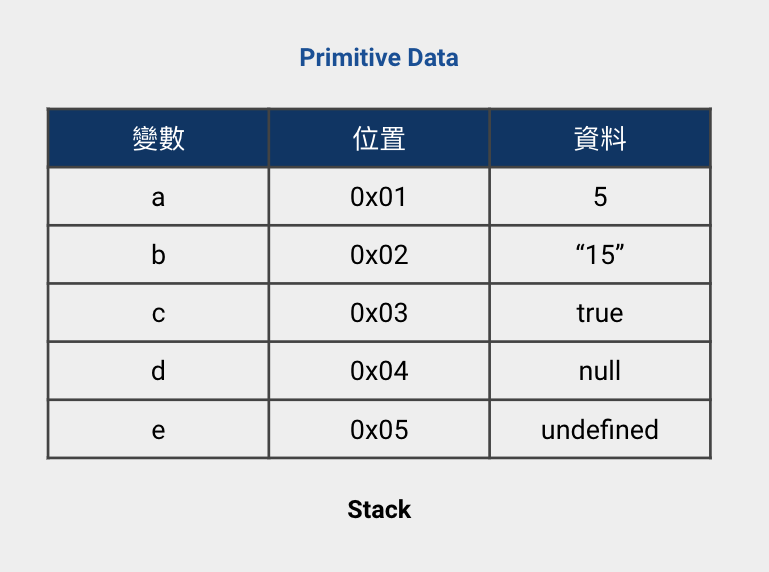`Stack` 是相對小但存取相對快的記憶體空間，變數會以變數表的概念儲存於其中，表中包含：「變數名稱」、「記憶體位置」、「資料的值」。

• 基本型別 (Primitive type)：在 `Stack` 中，會直接儲存資料值 (value)，如上面那張圖，都是 `Primitive type data` 的變數。
• 物件型別 (Object)：在 `Stack` 中，僅會儲存資料值所在的記憶體位置的地址 (address)，用以當作參考 (reference)，這個參考會指向 `Heap` 中的資料值，如下圖。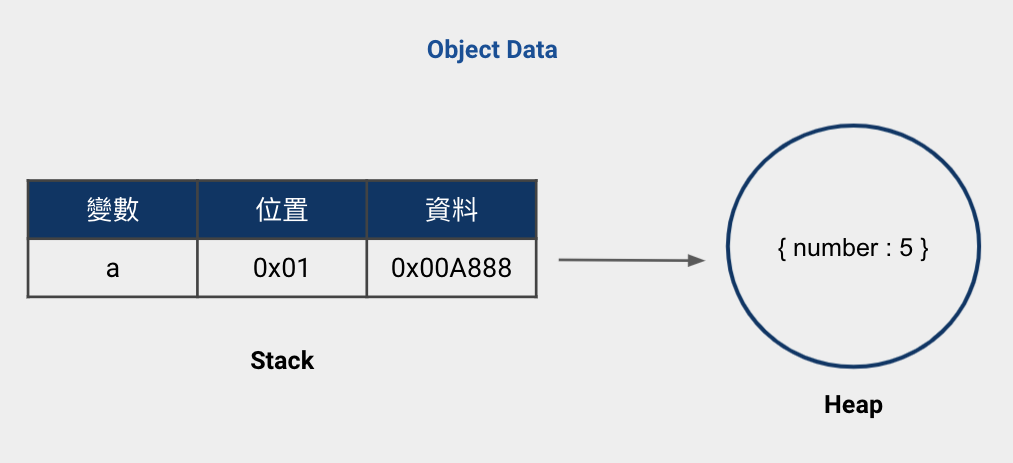`Heap` 相對於 `Stack` 是較大的記憶體空間，更適合儲存 `Object data` 這種較大的資料，當然相對存取會比較慢。

## 在記憶體中，複製變數的行為和結果

``````// Primitive type data 的複製
let a = 5;
let b = a; // 複製 a 的變數 b
``````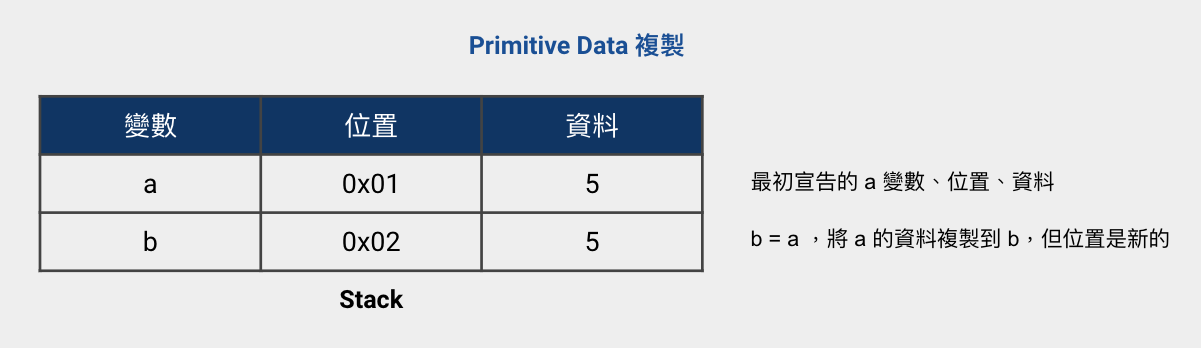``````// Object data 的複製
let a = { number: 5 };
let b = a; // 複製 a 的變數 b
``````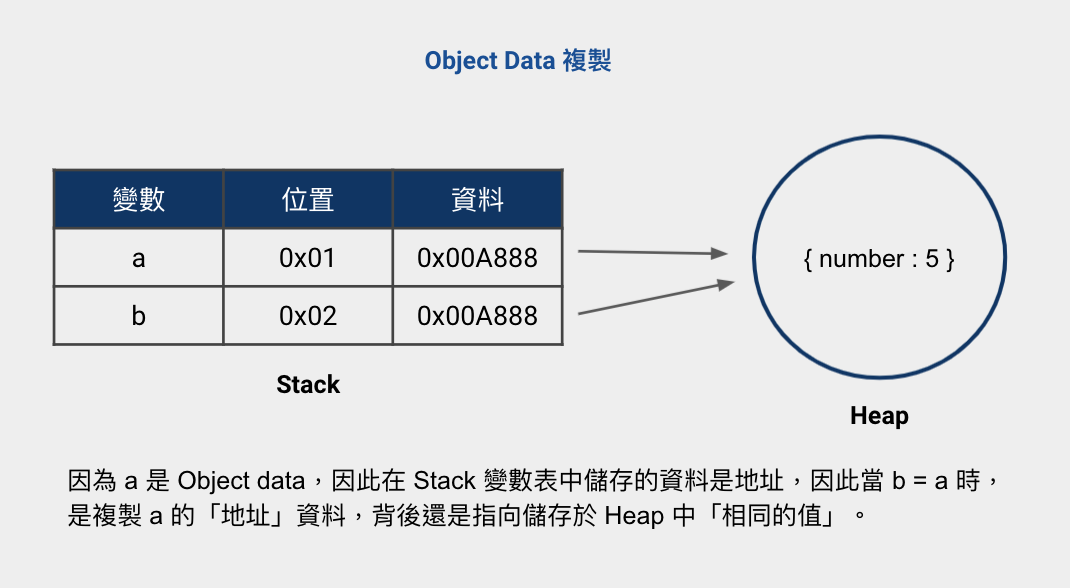• 基本型別 (Primitive type)：在複製變數時，會直接複製原始的值
• 物件型別 (Object)：在複製變數時，僅複製地址，而地址背後會指向相同的值

``````// Primitive type data 的複製
let a = 5;
let b = a;

console.log(a); // 5
console.log(b); // 5

a = 10;

console.log(a); // 10
console.log(b); // 5 => 沒有跟著 a 改變
``````

1. 宣告變數 `a`，並且將 `a` 賦值為 `5`，接著宣告變數 `b` 並且複製 `a` 變數，讓 `b = a`，這時候印出來的值都是 `5`
2. 將變數 `a` 重新賦值為 `10`，觀察看看是否會影響到複製的變數 `b`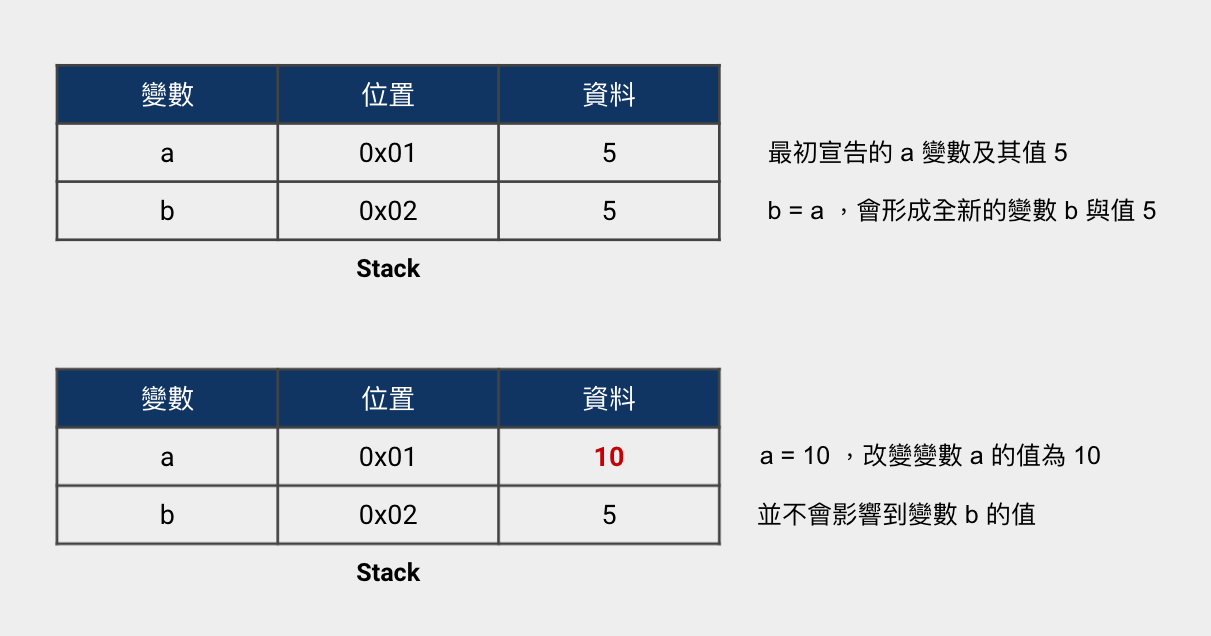``````// Object data 的複製
let a = { number: 5 };
let b = a;

console.log(a); // { number : 5 }
console.log(b); // { number : 5 }

a.number = 10;

console.log(a); // { number : 10 }
console.log(b); // { number : 10 } => 跟著 a 改變
``````

1. 宣告變數 `a`，並且將 `a` 賦值為物件 `{ number : 5}`，接著宣告變數 `b` 並且複製 `a` 變數，讓 `b = a`，這時候印出來的值都是 `{ number : 5}`
2. 利用 `a.number = 10` 將變數 `a` 改變為 `{ number : 10}`，觀察看看是否會影響到複製的變數 `b`• 當變數資料為 `Primitive type data` 時，在複製變數時，會完全複製一份新的「值 (value)」。
• 當變數資料為 `Object data` 時，在複製變數時，是複製一份新的「地址 (address)」，並非複製值 (value)，而相同的地址背後會指向同樣的值。

## 從 function 的傳遞參數，理解 pass by value

``````function test(primitiveData) {
primitiveData = primitiveData + 5;
console.log(primitiveData); // 10
}

let a = 5; // Primitive type data
test(a);

console.log(a); // 5 => 沒被改變
``````

1. 宣告 `function test(primitiveData)`
2. 宣告變數 `a` 賦值為基本型別的資料 `5`
3. `a` 丟進 `test` 函式中時，等同於是 `primitiveData` 複製 `a`，可以想成 `primitiveData = a`，產出函式中新的區域變數 `primitiveData`
4. 由於變數資料是基本型別，複製變數時是直接複製「值 (value)」，所以變數 `a` 與變數 `primitiveData` 擁有各自獨立的值
5. 因此改變任一變數的內容不會影響另一個變數，所以最後 `a` 印出依然不變，是原本的 `5`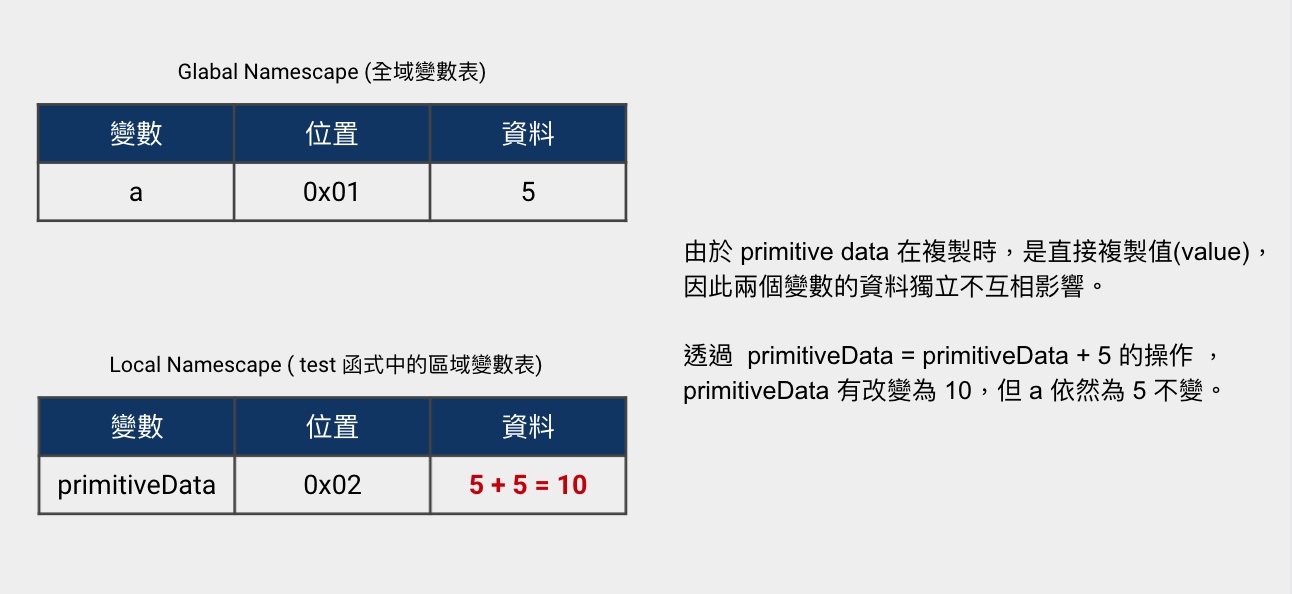`pass` 可以想成是傳遞函式參數的「傳遞」，`by value` 可以想成是傳遞變數時，是複製了傳遞進來的「值 (value)」。產生的結果就是函式內變數的值與傳入變數的值，各自獨立，不會互相影響。

`pass by value` 同時也可以稱之為 `call by value`，畢竟函式可以用呼叫的 (call)。

## 從 function 的傳遞參數，理解 pass by reference

``````function test(objectData) {
objectData.number = 10; // 改變物件內容
console.log(objectData); // { number: 10 }
}

let a = { number: 5 }; // Object data
test(a);

console.log(a); // { number: 10 } => 跟著改變
``````

1. 宣告 `function test(objectData)`
2. 宣告變數 `a` 賦值為物件型別的資料 `{ number : 5}`
3. `a` 丟進 `test` function 中，等同於是 `objectData` 複製 `a`，可以想成 `objectData = a`，形成函式中新的區域變數 `objectData`
4. 由於變數資料是物件型別，複製變數時是複製「地址 (address)」而非值，所以 `objectData``a` 擁有同樣的地址，指向同樣的值
5. 因此透過 `objectData.number = 10`，改變 `objectData` 的物件內容時，`a` 也同時被修改，最後印出的值是新的 `{ number: 10 }`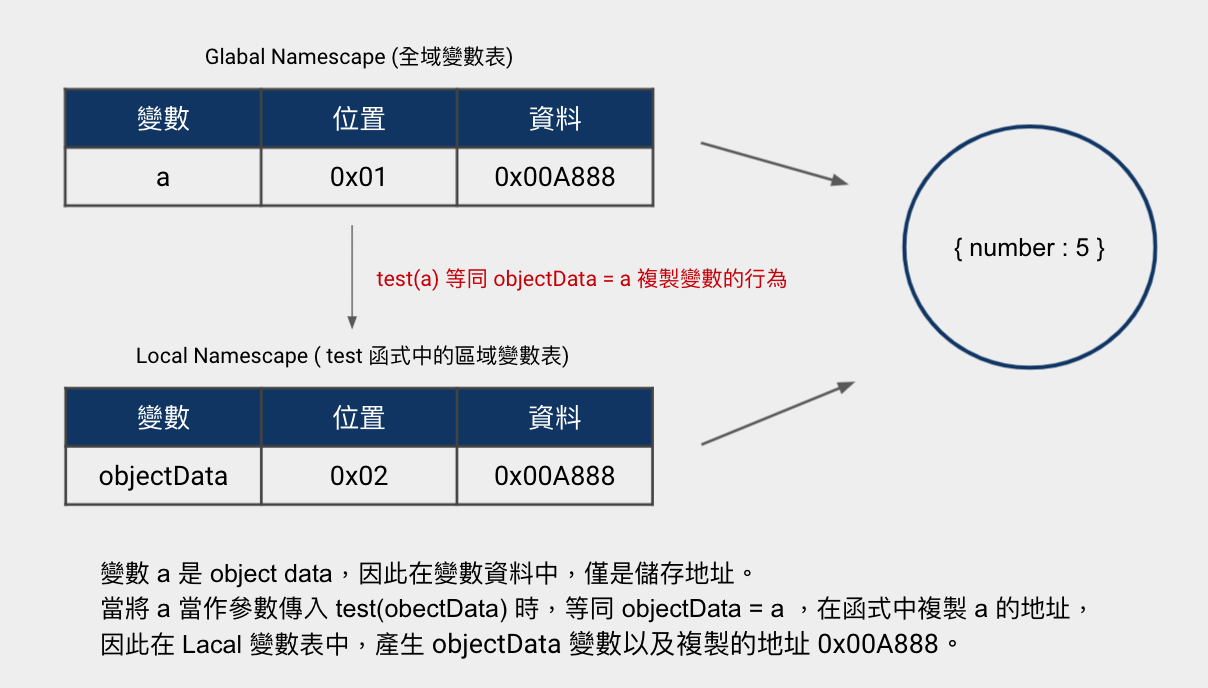`pass` 可以想成是傳遞函式參數的「傳遞」，`by reference` 則是指傳遞參數時，僅是複製了參數的「地址 (address)」作為真正值的參考座標 (reference)。產生的結果就是函式內變數的值與傳入變數的值，透過 `objectData.number` or `a.number` 改變物件內容時，是會互相影響的。

## 咦，怎麼又冒出個 pass by sharing ?

``````function test(objectData) {
objectData = { number: 10 }; // 物件重新賦值
console.log(objectData); // { number: 10 }
}

let a = { number: 5 }; // object data
test(a);

console.log(a); // { number: 5 } =>  那尼！居然沒跟著改變！
``````

1. 宣告 `function test(objectData)`
2. 宣告變數 `a` 賦值為物件型別的資料 `{ number : 5}`
3. `a` 丟進 `test` function 中，等同於是 `objectData` 複製 `a`，可以想成 `objectData = a`，形成函式中新的區域變數 `objectData`
4. 由於變數資料是物件型別，透過 `objectData = { number : 10 }` 重新賦值，此時產生一個新的地址對應新的物件值，objectData 會擁有新的地址，指向新的值
5. 因此 `a``objectData` 的地址不同，指向的值也不同，所以最後印出的 `a``{ number: 5 }`，沒有因為 `objectData` 重新賦值而被改變。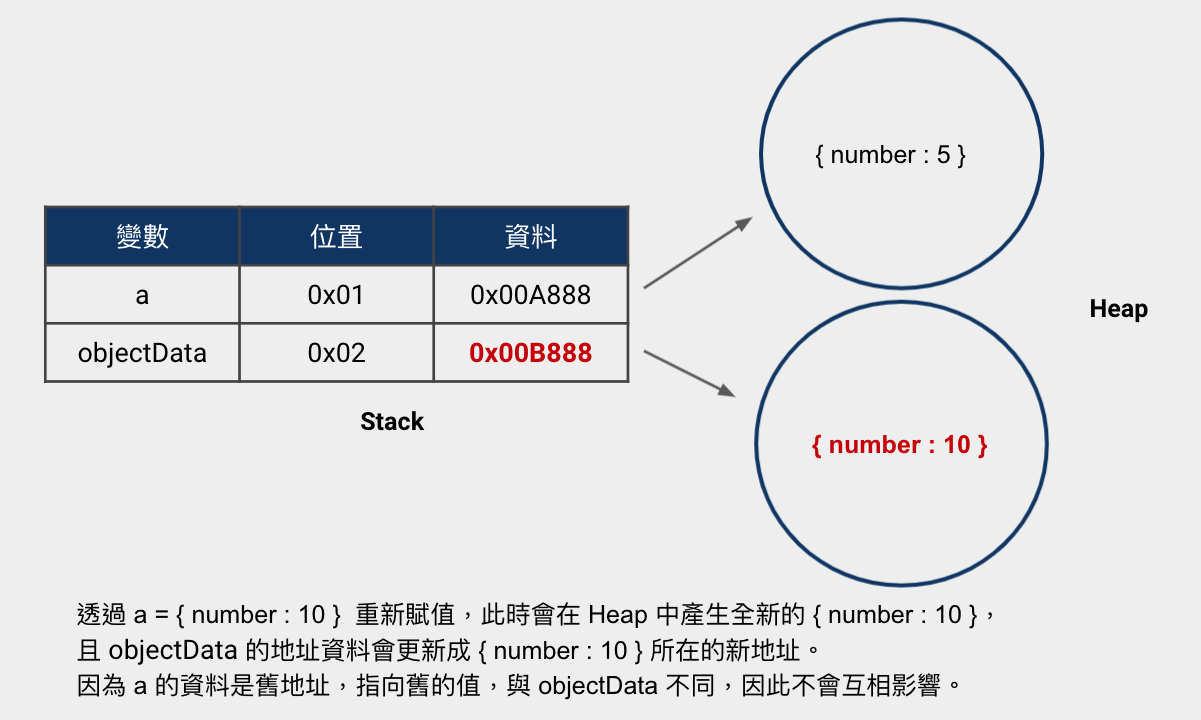• pass by reference 的概念 : 傳遞參數進函式後，透過 `object.number = 10` 改變內容，由於外部變數與內部變數的地址相同，指向同樣的值，因此會互相影響
• pass by value 的概念 : 傳遞參數進函式後，透過 `objectData = { number: 10 }` 重新賦值，此時會創建新的值與地址。由於外部變數與內部變數的地址不同，指向不同的值，因此並不會互相影響

• 如果遇到基本型別 (Primitive type)，原始變數「不會」跟著複製變數的改變而變，表現出的行為結果是 `pass by value`

``````function test(primitiveData) {
primitiveData = primitiveData + 5;
console.log(primitiveData); // 10
}

let a = 5; // Primitive type data
test(a);

console.log(a); // 5 => 沒被改變
``````
• 如果遇到物件型別 (Object)，且僅針對物件的內容做改變，原始變數「會」跟著複製變數的改變而變，表現出的行為結果是 `pass by reference`

``````function test(objectData) {
objectData.number = 10; // 改變物件內容
console.log(objectData); // { number: 10 }
}

let a = { number: 5 }; // Object data
test(a);
console.log(a); // { number: 10 } => 跟著改變
``````
• 如果遇到物件型別 (Object)，且對物件做重新賦值，原始變數「不會」跟著複製變數的改變而變，表現出的行為結果是 `pass by value`

``````function test(objectData) {
objectData = { number: 10 }; // 物件重新賦值
console.log(objectData); // { number: 10 }
}

let a = { number: 5 }; // object data
test(a);
console.log(a); // { number: 5 } => 沒被改變
``````

## 為什麼有 JavaScript 都是 pass by value 的說法 ?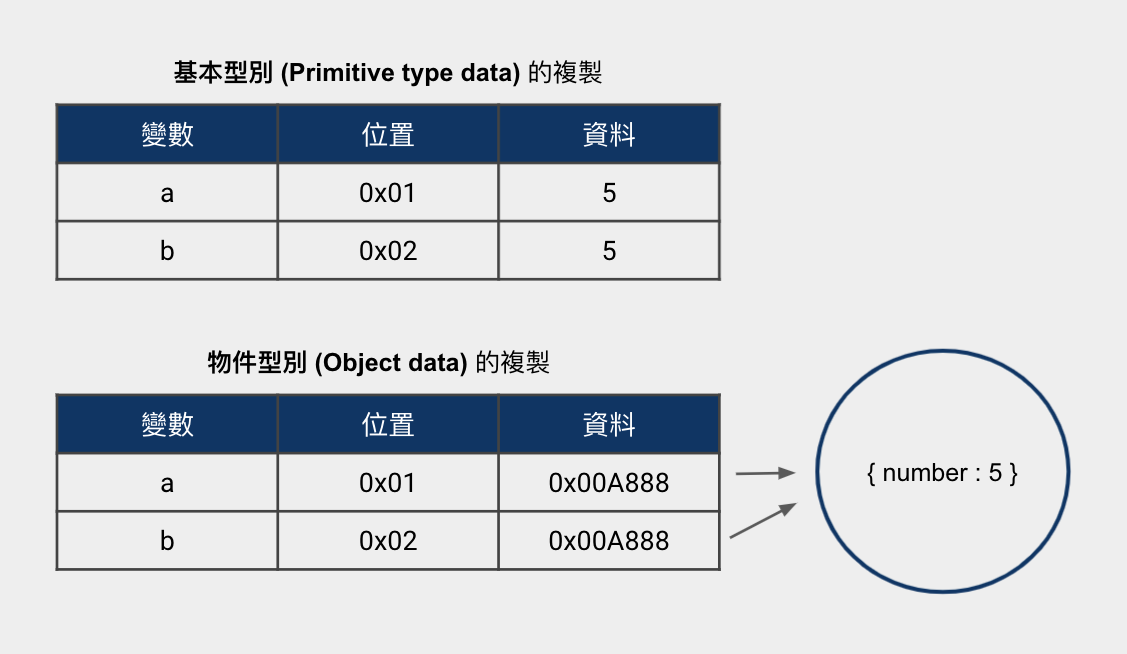## 總結：比起技術名詞，更該在意「複製變數的過程是如何運行的」

• JavaScript 中，`Primitive type` 的變數資料是 `pass by value`，而 `Object` 的變數資料是 `pass by sharing`
• JavaScript 都是 `pass by value`

1. 如果是 `Primitive type` 的變數值，例如：`const a = 5`，那麼複製變數時，是會複製「原始的值 (value)」，因此原始變數與複製變數「不會」互相影響。
2. 如果是 `Object` 的變數值，例如：`const a = { number : 5 }`，那麼複製變數時，是會複製「地址 (address)」，當相同地址指向相同的值時，原始變數與複製變數「會」互相影響。但需要注意「重新賦值」的行為，會產生全新的地址和全新的值。
3. 將外部變數當作參數，傳入 function 時，就代表在 funcion 作用域中，複製外部變數，產生新的內部變數。

``````/*** 基本型別 ***/
function test(primitiveData) {
primitiveData = primitiveData + 5;
console.log(primitiveData); // 10
}

let a = 5; // primitive data
test(a);
console.log(a); // 5 => 沒被改變

/*** 物件型別之改變內容 ***/
function test(objectData) {
objectData.number = 10; // 改變物件內容，無重新賦值
console.log(objectData); // { number: 10 }
}

let a = { number: 5 }; // object data
test(a);
console.log(a); // { number: 10 } => 跟著改變

/*** 物件型別之重新賦值 ***/
function test(objectData) {
objectData = { number: 10 }; // 物件重新賦值
console.log(objectData); // { number: 10 }
}

let a = { number: 5 }; // object data
test(a);

console.log(a); // { number: 5 } => 沒被改變
``````

「在《松本行弘的程式設計世界》的〈語彙與共通語言的重要性〉這篇文章中，作者談到，為某個概念決定適當的名詞，目的是在設計時能有共同的語彙，也能讓開發者意識到它們的存在，這才是名詞存在的真正意義。」—《技術名詞紛爭多

「技術名詞是為了描述概念而存在，而不是概念為了技術名詞而存在。最重要的是背後期望表達的概念，也就是體現出來的『行為』。」—《你不可不知的 JavaScript 二三事#Day26：程式界的哈姆雷特 — Pass by value, or Pass by reference？